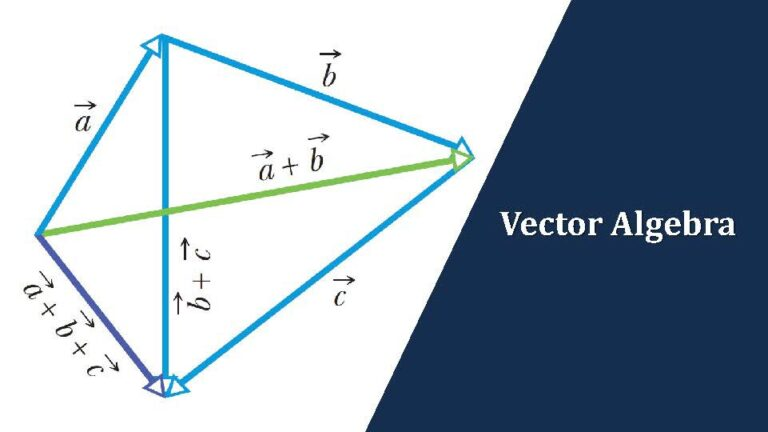IIT & JEE Main Physics | Kinematics | VectorsIn the recent years, in both JEE Advanced and JEE Main physics kinematics section, we are seeing questions on vectors. So pay a close attention to the question types below.

Note that the scope of such questions is limited in physics paper in these exams as a more detailed treatment of this topic is under the scope of mathematics. This is reflected in the fact that there are only few types of questions that can be expected on this topic in physics paper

Week 2 – Vectors (June 9 – June 15, 2021)

IIT & JEE Main Physics Kinematics | Question Types on Vectors

There are only 3 different types of questions you can expect on vectors in JEE Mains and JEE Advanced.

In JEE Mains there seems to be a greater focus on question type where in students will have to calculate angle between the vectors while in JEE Advanced you can expect interesting questions from the question type named ‘General Vector Algebra’

So let’s get into these question types

Question type 10:

Given coordinates of two point, find the vector joining those two points

Question Type 11:

Here you will be asked to find angle between two vectors given some relation between the two vectors. Here the two vector algebra operations that come in handy are

(1) $\overrightarrow{A}.\overrightarrow{B}=$ $AB \cos \theta$

(2) $|\overrightarrow{A}+\overrightarrow{B}|^2=$ $(\overrightarrow{A}+\overrightarrow{B}).$$(\overrightarrow{A}+\overrightarrow{B}).$

Question Type 12:

Questions involving use of vector algebra with vectors either given in unit vector notation or in graphical representation

That concludes a brief review of question types on vectors in IIT and JEE Main physics kinematics